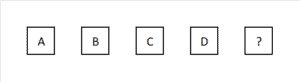# Abstract reasoning: number sequence

• Start practicing immediately
• Compare your score to the recommended pass score
• Courses followed by quizzes
• Practice quizzes similar to real tests

Hellotest offers the best preparation for all types of aptitude tests. With over 10.000 questions with explanations and clear courses, you will optimally prepare for your test.

At Hellotest you will find extensive guides on which tests you have to prepare for your specific test. You will spend less time preparing and still be better prepared for your test.

## Practice number sequence aptitude tests

Start practicing for number sequence aptitude tests with our custom made practice package:

• Clear lessons with explanation.
• Test quizzes that resemble true aptitude tests.

## What are number sequences?

Number sequences is part of numerical reasoning. Number sequences are often used in assessments. The idea of a number series is that you get to see a few numbers and then have to determine the last number. To determine the last number, you will often have to perform simple calculations.

The calculations that you must perform are:

• subtractions,
• multiplying,
• dividing by,
• fractures,
• percentages,
• decimals,
• powers.

The tricky thing about number series is that there are even morevariations:

• skip numbers,
• increasing or decreasing changes,
• series of calculations.

## Example of an easy number sequenceA number sequence consists of a series of numbers where you have to determine the last one.

Unique to these number series is that these have two outcomes. So you not only have to calculate the next but also the following number. This makes it virtually impossible to gamble on the test.

The tricky thing about these numbers is that you get different types of calculations mixed together. For example, you will come across a combination of addition and subtraction. But you can also come across a combination of addition and division.

## Double mutation number sequences

Sometimes, number sequences have double mutations. So, the addition is not simply +1 +1 +1 +1 +1. Instead it is +1 +2 +3 +4 +5. So there is another underlying change, again +1 +1 +1 +1.

You will find that these double mutations can be quite difficult. For instance, when the change becomes negative or positive during the calculations: +1 +0 -1 -2 -3. Practicing these types of number sequences makes sure you will be better prepared for your test.

### Specific

Specific practice material for all major test publishers.

### Quality

The best way to practice according to recruiting specialists.

### Instant

Start practicing immediately, no waiting time.